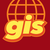# Create feature layer from geometry

534
0
10-24-2018 11:38 PMNew Contributor III

Hi

I looked extensively in this forum but I cannot get to decipher the answer. If I create a polygon (this is from an example in here) and want to make that into a feature layer, how can this be done? This would be useful for me to do. So, say I create a polygon from bufferin an initial point:

import arcpy,json

x = 116.6735507

y = -31.28888386

point = arcpy.Point(float(x),float(y))

sr = arcpy.SpatialReference(3857)

ptGeometry = arcpy.PointGeometry(point,sr)

length = 1

buff_geometry = ptGeometry.buffer(length)

densify = buff_geometry.densify('DISTANCE',1,1)

shape = densify.JSON